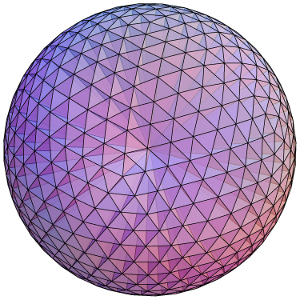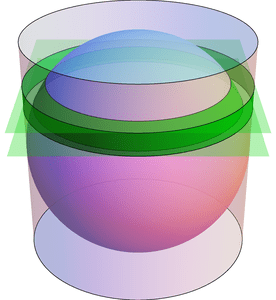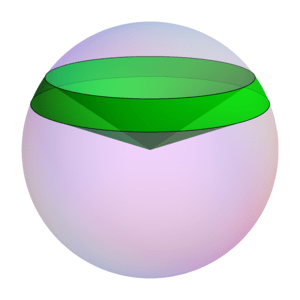# Spherical Surfaces and Hat Boxes

To round off our series on round objects (see the first and second posts), let’s compute the sphere’s surface area. We can compute this in the same way we related the area and circumference of a circle two weeks ago. Approximate the surface of the sphere with lots of small triangles, and connect these to the center of the sphere to create lots of triangular pyramids. Each pyramid has volume $$\frac{1}{3}(\text{area of base})(\text{height})$$, where the heights are all nearly $$r$$ and the base areas add to approximately the surface area. By using more and smaller triangles these approximations get better and better, so the volume of the sphere is $$\frac{4}{3}\pi r^3 = \frac{1}{3}(\text{surface area})\cdot r,$$ meaning the surface area is $$4\pi r^2$$. (This and previous arguments can be made precise with the modern language of integral calculus.)Dividing a sphere into many pyramids connected to the center allows us to relate the sphere's surface area and volume.

Here’s an elegant way to rephrase this result: The surface area of a sphere is equal to the area of the curved portion of a cylinder that exactly encloses the sphere. In fact, something very surprising happens here!:

Archimedes’ Hat-Box Theorem: If we draw any two horizontal planes as shown below, then the portions of the sphere and the cylinder between the two planes have the same surface area.Any two horizontal planes cut off a band on the sphere and another band on the enclosing cylinder. Archimedes' Hat-Box Theorem says that these bands have the same area. (The planes shown here have heights $$0.4\cdot r$$ and $$0.6\cdot r$$ above the equator.)

We can prove this with (all!) the methods in the last few posts; here’s a quick sketch. To compute the area of the “spherical band” (usually called a spherical zone), first consider the solid spherical sector formed by joining the spherical zone to the center:The Hat-Box theorem can be proved by relating the area of the spherical zone to the volume of this spherical sector.

By dividing this into lots of triangular pyramids as we did with the sphere above, we can compute the area of the spherical zone by instead computing the sector’s volume. This volume can be computed by breaking it into three parts: two cones and the spherical segment between the two planes (on the left of the next figure). Compute the volume of the spherical segment by comparing (via Cavalieri’s Principle) to the corresponding part of the vase (from the previous post), which can be expressed with just cylinders and cones.

See if you can fill in the details!

# Archimedes’ Circular Reasoning

Every geometry textbook has formulas for the circumference ($$C = 2 \pi r$$) and area ($$A = \pi r^2$$) of a circle. But where do these come from? How can we prove them?

Well, the first is more a definition than a theorem: the number $$\pi$$ is usually defined as the ratio of a circle’s circumference to its diameter: $$\pi = C/(2r)$$. Armed with this, we can compute the area of a circle. Archimedes’ idea (in 260 BCE) was to approximate this area by looking at regular $$n$$-sided polygons drawn inside and outside the circle, as in the diagram below. Increasing $$n$$ gives better and better approximations to the area.The n-sided regular polygons inside (blue) and outside (red) a circle can be used to approximate the area of the circle. Shown here for n=12.

Look first at the inner polygon. Its perimeter is slightly less than the circle’s circumference, $$C = 2 \pi r$$, and the height of each triangle is slightly less than $$r$$. So when reassembled as shown, the triangles form a rectangle whose area is just under $$C/2\cdot r = \pi r^2$$. Likewise, the outer polygon has area just larger than $$\pi r^2$$. As $$n$$ gets larger, these two bounds get closer and closer to $$\pi r^2$$, which is therefore the circle’s area.

Archimedes used this same idea to approximate the number $$\pi$$. Not only was he working by hand, but the notion of “square root” was not yet understood well enough to compute with. Nevertheless, he was amazingly able to use 96-sided polygons to approximate the circle! His computation included impressive dexterity with fractions: for example, instead of being able to use $$\sqrt{3}$$ directly, he had to use the (very close!) approximation $$\sqrt{3} > 265/153$$. In the end, he obtained the bounds $$3\frac{10}{71} < \pi < 3\frac{1}{7}$$, which are accurate to within 0.0013, or about .04%. (In fact, he proved the slightly stronger but uglier bounds $$3\frac{1137}{8069} < \pi < 3\frac{1335}{9347}$$. See this translation and exposition for more information on Archimedes’ methods.)

These ideas can be pushed further. Focus on a circle with radius 1. The area of the regular $$n$$-sided polygon inscribed in this circle can be used as an approximation for the circle’s area, namely $$\pi$$. This polygon has area $$A_n = n/2 \cdot \sin(360/n)$$ (prove this!). What happens when we double the number of sides? The approximation changes by a factor of $$\frac{A_{2n}}{A_n} = \frac{2\sin(180/n)}{\sin(360/n)} = \frac{1}{\cos(180/n)}.$$ Starting from $$A_4 = 2$$, we can use the above formula to compute $$A_8,A_{16},A_{32},\ldots$$, and in the limit we find that $$\pi = \frac{2}{\cos(180/4)\cdot\cos(180/8)\cdot\cos(180/16)\cdots}.$$ Finally, recalling that $$\cos(180/4) = \cos(45) = \sqrt{\frac{1}{2}}$$ and $$\cos(\theta/2) = \sqrt{\frac{1}{2}(1+\cos\theta)}$$ (whenever $$\cos(\theta/2) \ge 0$$), we can rearrange this into the fun infinite product $$\frac{2}{\pi} = \sqrt{\frac{1}{2}} \cdot \sqrt{\frac{1}{2}+\frac{1}{2}\sqrt{\frac{1}{2}}} \cdot \sqrt{\frac{1}{2}+\frac{1}{2}\sqrt{\frac{1}{2}+\frac{1}{2}\sqrt{\frac{1}{2}}}} \cdots$$ (which I found at Mathworld). (It’s ironic that this formula for a circle uses so many square roots!)